# CBSE Question Paper 2008 class 12 Mathematics## myCBSEguide App

Complete Guide for CBSE Students

NCERT Solutions, NCERT Exemplars, Revison Notes, Free Videos, CBSE Papers, MCQ Tests & more.

CBSE Question Paper 2008 class 12 Mathematics conducted by Central Board of Secondary Education, New Delhi in the month of March 2008. CBSE previous year question papers with solution are available in myCBSEguide mobile app and cbse guide website. The Best CBSE App for students and teachers is myCBSEguide which provides complete study material and practice papers to cbse schools in India and abroad.

CBSE Question Paper 2008 class 12 Mathematics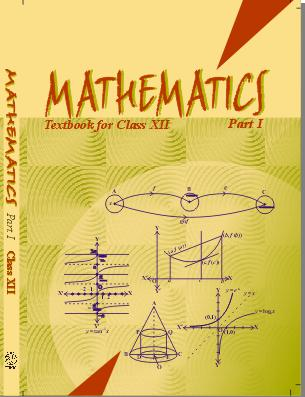## Class 12 Mathematics list of chapters

1. Relations and Functions
2. Inverse Trigonometric Functions
3. Matrices
4. Determinants
5. Continuity and Differentiability
6. Application of Derivatives
7. Integrals
8. Application of Integrals
9. Differential Equations
10. Vector Algebra
11. Three Dimensional Geometry
12. Linear Programming
13. Probability

## CBSE Question Paper 2008 class 12 Mathematics

### General Instruction:

• All question are compulsory.
• The question paper consists of 29 questions divided into three section A, B and C. Section A comprises of 10 questions of one mark each, Section B comprises of 12 questions of four marks each and Section C comprises of 7 questions of six marks each.
• All question in Section A are to be answered in one word, one sentence or as per the exact requirement of the questions.
• There is no overall choice. However, internal choice has been provided in 4 questions of four marks each and 2 questions of six marks each. You have to attempt only one of the alternatives in all such questions.
• Use of calculators is not permitted.

### Section A

1. Evaluate: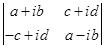2. Find the value of x and y if: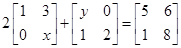3. If f(x) = x + 7 and g(x) = x – 7, x∈x∈ R, find (fog) (7)

4. Evaluate: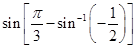5. Evaluate: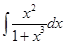6. Fined the co-factor a12 in the following: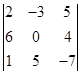7. For what value of λ are the vectors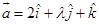and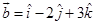8. Find the angle between the vectors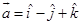and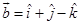9. Find a unit vector in the direction of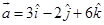10. Evaluate: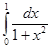### Section B

11. Solve the following differential equation: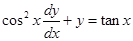12. Solve the followng differential euation: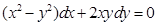Give that y =when x = 1

### OR

Solve the following differential equation: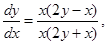if y = 1 when x = 1

13. Find trhe shortest distance between the following lines: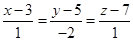and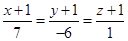### OR

Find the point on the line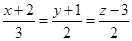at a distance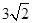from the point (1, 2, 3)

14. A pair of dice is thrown 4 times. If getting a doublet is considered a success, find the probability distribution of number of successes.

15. if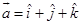and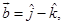find a vector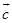such that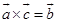and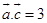OR

If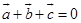and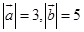and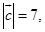show that the angle between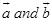is 60°.

16. Let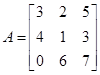, Express A as sum of two matrices such that one is symmetric and the other is skew symmetric.

### OR

If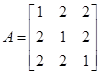verify that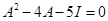17. For What value of k is the following function continuous at x = 2 ?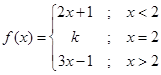18. Find the equation of tangent to the curve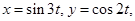at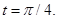19. (i) Is the binary operation *, defined on set N, given by a*b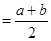for all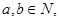commutative ?

(ii) Is the above binary operation * associative ?

20. Solve for x :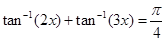21. Evaluate: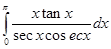22. If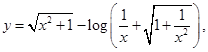find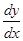### Section C

23. Using properties of determinants, prove the following: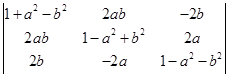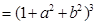24. Evaluate: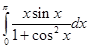25. Using integration, find the area of the region enclosed between the circles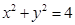and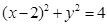26. An insurance company insured 2000 scooter drivers, 4000 car drivers and 6000 truck drivers. The probability of an accident involving a scooter, a car and a truck are 0.01, 0.03 and 0.15 respectively. One of the insured persons meets with an accident. What is the probability that he is a scooter driver.

27. Find the equation of the plane passing through the point (-1, -1, 2) and perpendicular to each of the following planes: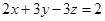and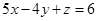### OR

Find the equation of the plane passing the points (3, 4, 1) and (0, 1, 0) and parallel to the line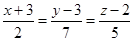28. A factory owner purchases two types of machines, A and B for his factory.
The requirements and the limitations for the machines are follows:

 Machine Area occupied Labour force Daily output (in units) AB 1000 m21200 m2 12 men8 men 6040

He has maximum are 9000 m2 available, and 72 skilled labourers who can operate both the machines. How many machines of each type should he buy to maximize the daily output ?

29. Show that the rectangle of maximum area that can be inscribed in a circle is a square.

### OR

Show that the height of the cylinder of maximum volume that can be inscribed in a cone of height h is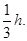These are questions only. To view and download complete question paper with solution install myCBSEguide App from google play store or login to our student dashboard.

## Previous Question Paper for Class 12 Mathematics

Download class 12 Mathematics question paper with solution from best CBSE App the myCBSEguide. CBSE class 12 Mathematics question paper 2012 in PDF format with solution will help you to understand the latest question paper pattern and marking scheme of the CBSE board examination. You will get to know the difficulty level of the question paper.

## Last Year Question Paper 2008

CBSE question papers 2018, 2017, 2016, 2015, 2014, 2013, 2012, 2011, 2010, 209, 2008, 2007, 2006, 2005 and so on for all the subjects are available under this download link. Practicing real question paper certainly helps students to get confidence and improve performance in weak areas.

To download CBSE Question Paper 2012 class 12 Accountancy, Chemistry, Physics, History, Political Science, Economics, Geography, Computer Science, Home Science, Accountancy, Business Studies and Home Science; do check myCBSEguide app or website. myCBSEguide provides sample papers with solution, test papers for chapter-wise practice, NCERT solutions, NCERT Exemplar solutions, quick revision notes for ready reference, CBSE guess papers and CBSE important question papers. Sample Paper all are made available through the best app for CBSE students and myCBSEguide website.## Test Generator

Create Papers with your Name & Logo

### 1 thought on “CBSE Question Paper 2008 class 12 Mathematics”

1. Really very appreciable blog.Thanks for sharing valuable content